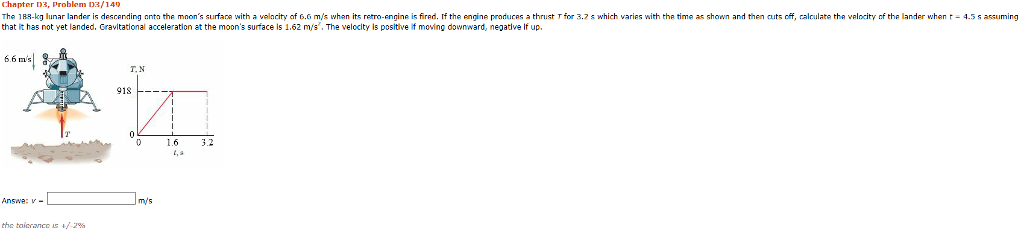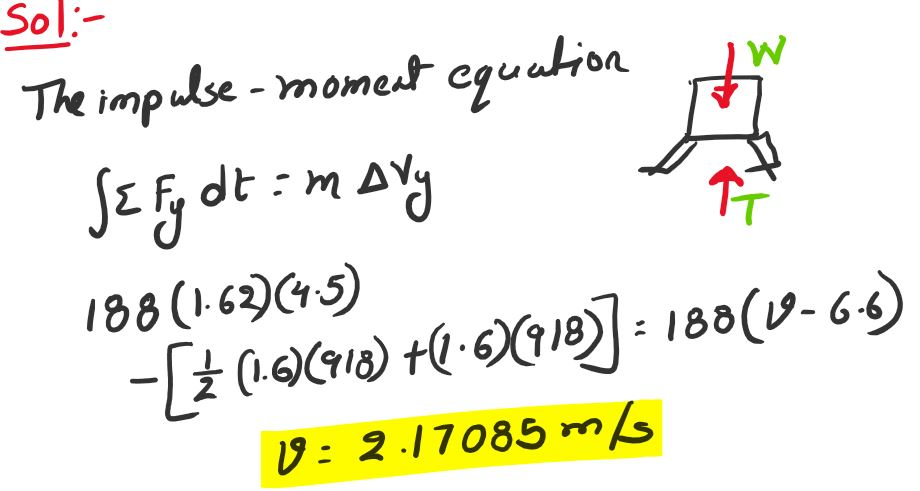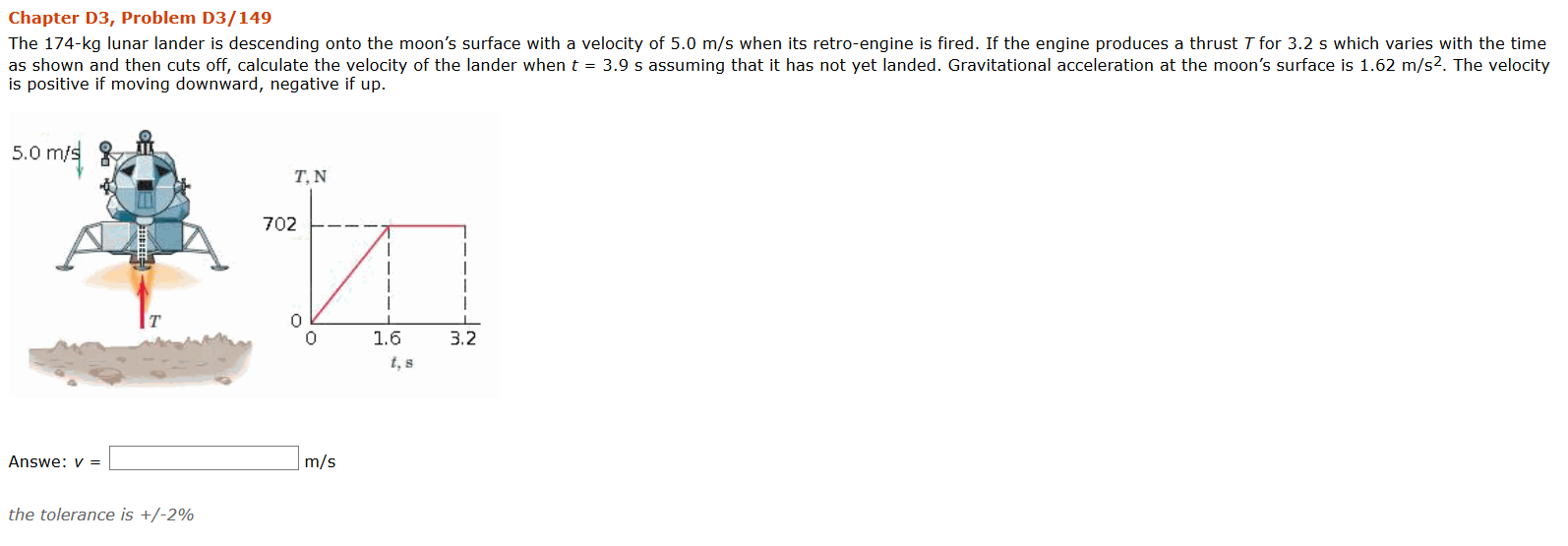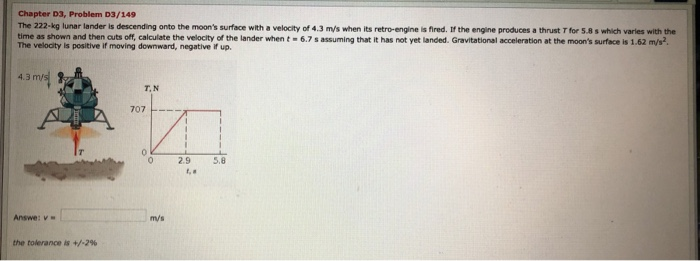Question

# Chapter D3, Problem D3/140 The 188 g unar lander is descending nnta he mnon's surfan with...Chapter D3, Problem D3/140 The 188 g unar lander is descending nnta he mnon's surfan with a velac y of 6 m 尿when its re o engine ts fired f the engine producas a thrust T or 3.2昴which varies with the that it has not yet landed, Gravitational acceleration at the moon's surface is 1.62 m/s, The velocity is positive if moving downward, negative If up, me as shown and then cuts o calculate the velocity the lander when t 4.5 s assuming 6.6 m's T.N 918 1.6 3.2 Answe: - mys the tolerance t/ 29#### Earn Coins

Coins can be redeemed for fabulous gifts.

Similar Homework Help Questions
• ### Chapter D3, Problem D3/149 The 174-kg lunar lander is descending onto the moon's surface with a...Chapter D3, Problem D3/149 The 174-kg lunar lander is descending onto the moon's surface with a velocity of 5.0 m/s when its retro-engine is fired. If the engine produces a thrust T for 3.2 s which varies with the time as shown and then cuts off, calculate the velocity of the lander when t = 3.9 s assuming that it has not yet landed. Gravitational acceleration at the moon's surface is 1.62 m/s2. The velocity is positive if moving downward,...

• ### Chapter D3, Problem 03/149 The 222-kg lunar lander is descending onto the moon's surface with a...Chapter D3, Problem 03/149 The 222-kg lunar lander is descending onto the moon's surface with a velocity of 4.3 m/s when its retro-engine is fired. If the engine produces a thrust T for 5.8 s which varies with the time as shown and then cuts off, calculate the velocity of the lander when t - 6.7 s assuming that it has not yet landed. Gravitational acceleration at the moon's surface is 1.62 m/s? The velocity is positive if moving downward,...